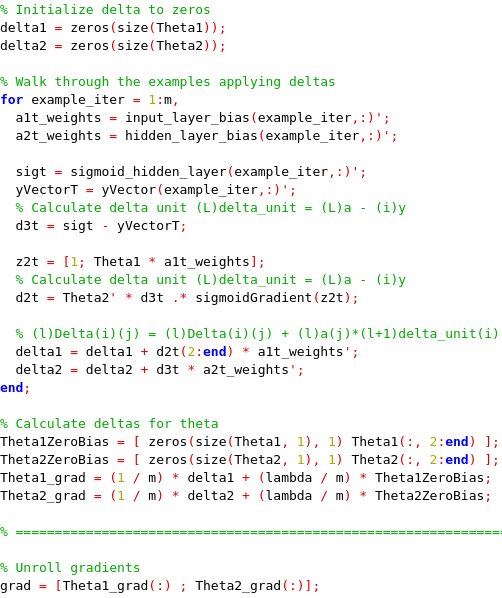# IntroductionFirst of all, let me tell you this is part of the Coursera Machine Learning course. Here I will show how to implement a Neural Network Backpropation in Octave. I know there are already several options to do this in a very high level (TensorFlow), just focusing on inputs and outputs, but I would like to understand all the matrix and statistical fundamentals to later be able to choose the right strategies in high level programs. Backpropagation algorithm is a guided learning algorithm, i.e., we need to traning the Neural Network with real outputs in order to obtain good predictions.

# Neural network design

We are going to design a Neural Network that will be able to recognize pictures with numbers, similar to captchas, but simpler. In this case these are the parameters:

• 3 layers: input layer, hidden layer and output layer
• Input layer: 400 input units, each images is a 20x20 pixels
• Hidden layer: 25 units
• Outputs layer: 10 units, from zero to nine
• We are gonna use backpropagation algorithm
• We have 5000 training examples

Once we have this we have to focus in the implementation: that will be valid for this architecture, 3 layers, applied for other kind of problems or classifiers.

# Neural Network Implementation

As you probably know machine learning, although I'm not an expert, is based on optimization, i.e., we are always trying to optimize the system, the prediction. If we remember how to optimize, or at least what we know about optimize from School, it was related with the derivate of a function and yes, that's what we apply here too. We now apply the partial derivatives of the functions to optimize them, to find where the cost of the function is zero by modifying the weights of the funcions, the Theeta's (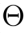). That's what happens in Neural Networks, we are trying to minimize the cost.

## Cost function

In order to minimize the cost, we first need to know the cost. The cost in a Feed Forward Neural Network is givin by the next formula: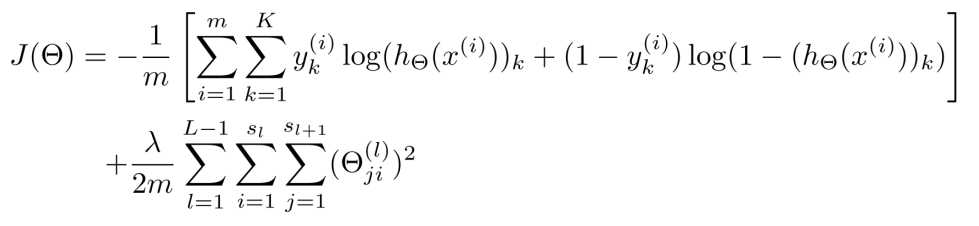where:

• x is the input
• y is the output of the training example
• h is the sigmoid function
• m is the number of examples, 5000 in our case
• L is the number of layer, 3 in our case
• K is the number of ouputs, 10 in our case [0-9]
• s is the number of units of layer l

So, let's start our implementation in Octave by adding our bias column: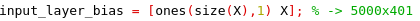Then, we calculate the sigmoid of this input layer: h(x)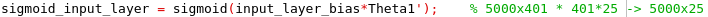We continue processing the Feed Forward in the hidden layer, adding also the bias input: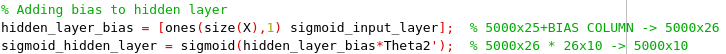We also have to generate a vector in which its index position corresponds to the actual result: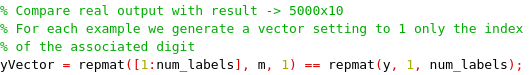Now we are able to calculate the partial costs, this look familiar with the formula above. It's really important to understand this, this means if the cost is close to real result it has a low cost and vice-versa.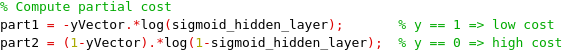We almost have it, we need just to add the regularization part (second line of the formula):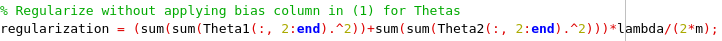And yes, we finally calculate the cost applying each part calculated above: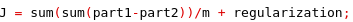Pretty easy, isn't it? Ok, so now we are able to calculate the cost for a given example, that sounds good but here there is not any learning yet. We are just calculating for each example how far or close are we to make a good prediction with the current weights. Image a plot where for each example we identify if the J result is giving a good prediction or not, that's what we are doing for now.

As we said before, there is several ways to minimize the cost, one of the is the gradient computation. There are a lot of maths behind this, but the idea is, we want to modify the weights to make good predictions for all the examples given. So the partial derivatives of the Cost function, J, give we have to apply the Deltas of the real result from the output to the input. In logistic regression as we have only one layer we do not need to perform any back propagation, but here we propagate the error (output - y) from the output to the input backwards (back propagation). By the propagation of that delta errors and applying them to the weights we are going to learn, we are adjusting the weights a litlle based on each example.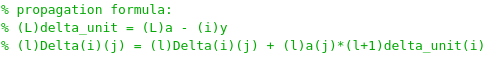where:

• l is the layer
• j is the

In our case, since we have two Theeta vectors we have to calculate to Delta vectors. Focusing the Delta vectors calc in one training example

We first obtain the weights of a particular example: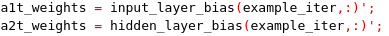We also will need to obtain the output example of the last layer and the vector real result, the iVector for that example: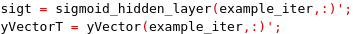Perfect, we now are able to calculate the Delta between the real result and the output in the last layer: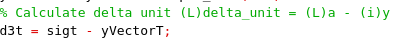Let'ts back-propagate the delta error to the previous layer: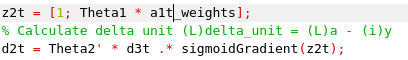Finally, calculating the Deltas for the two weights vectors, we obtaing the specific ajustment for this example. We are learning here, now the neural network is better recognizing inputs like this example: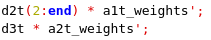Putting altogether, for m training examples: